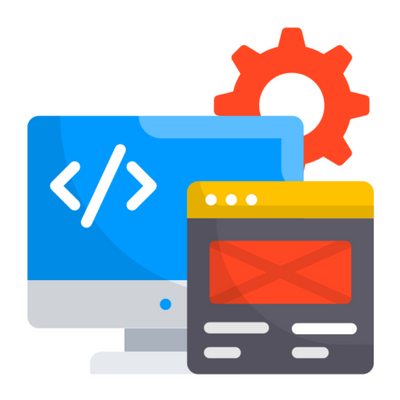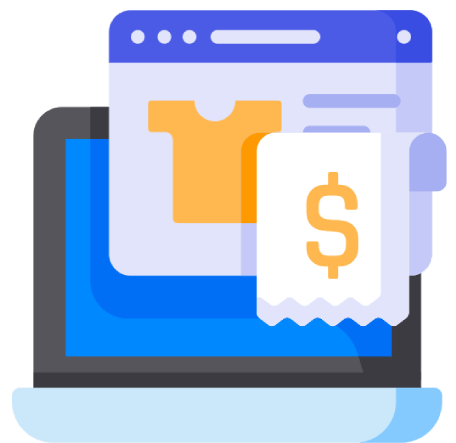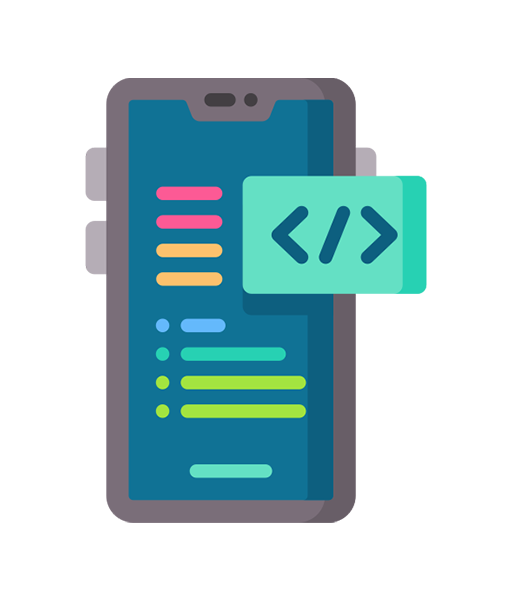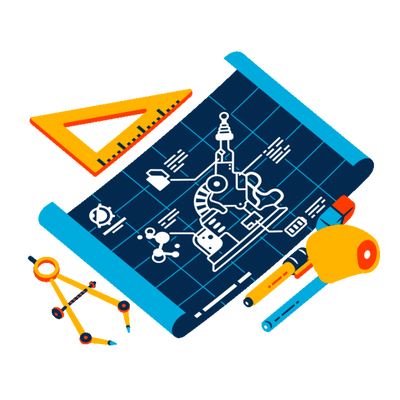## OUR EXPERTISE

D i g i t a l   S o l u t i o n s   T o   S c a l e   U p   Y o u r   B u s i n e s s
D i g i t a l   S o l u t i o n s   T o   S c a l e   U p   Y o u r   B u s i n e s s
D i g i t a l   S o l u t i o n s   T o   S c a l e   U p Y o u r   B u s i n e s s
D i g i t a l   S o l u t i o n s   T o   S c a l e   U p Y o u r   B u s i n e s s
D i g i t a l   S o l u t i o n s   T o   S c a l e U p   Y o u r   B u s i n e s s
D i g i t a l   S o l u t i o n s   T o   S c a l e U p   Y o u r   B u s i n e s s
D i g i t a l   S o l u t i o n s T o   S c a l e   U p   Y o u r B u s i n e s s
D i g i t a l   S o l u t i o n s T o   S c a l e   U p   Y o u r B u s i n e s s## Website Development## CRM & E-Commerce## Mobile App Development## Graphic Design & Animation## WORK WITH US

L e t ' s   K n o w   Y o u   B e t t e r
L e t ' s   K n o w   Y o u   B e t t e r
L e t ' s   K n o w   Y o u   B e t t e r
L e t ' s   K n o w   Y o u   B e t t e r

All * marked fields are mandatory